## Introduction

Polar molecules1,2,3,4,5,6 present a flexible platform for the exploration of quantum magnetism in many-body systems due to their strong and long-range dipole–dipole interactions and their rich internal structure of rotational levels. A few isolated rotational levels of a molecule represent an effective spin degree of freedom. Net spin–spin couplings can directly be generated by dipolar interactions even in frozen molecule arrays. Recent experiments7,8 with molecules pinned in a deep optical lattice have demonstrated dipolar spin-exchange coupling. The anisotropic dipole–dipole interaction can also couple the spin degrees of freedom to the orbital motion of the molecules. Signatures of this type of coupling have been recently reported experimentally in bosonic magnetic atoms9,10, and have been noted for their potential to generate topological phases11,12,13,14. All previously predicted phenomena were limited to zero-dimensional systems15 or weakly interacting bosonic systems at zero temperature or were tailored to particular experimental set-ups16,17,18,19,20, requiring, for example, complicated dressing techniques12,13.

Here we demonstrate that an effective spin–orbit coupling (SOC) is inherent in the excitations of any two-dimensional (2D) system of polar molecules with a pair of degenerate N=1 rotational levels. These excitations, which we call chirons, are characterized by a non-trivial Berry phase 2π. The same Berry phase is responsible for, for example, an unconventional quantum Hall effect in bilayer graphene21,22. Remarkably, in our system, SOC emerges due to interactions rather than being a single-particle effect, which adds significant richness to the physics and removes the fundamental limitations imposed by spontaneous emission present when single-particle SOC is artificially generated by light11,23,24,25,26. We present ways to detect chirality and Berry phase, for instance, by exciting rotational degrees of freedom in a finite spatial region. Generically, this leads to two fronts of spin and density currents, corresponding to the two branches of the chiron spectrum. The Berry phase 2π manifests itself in the d-wave symmetry cos(2φ) of the spin projection onto the plane, where φ is defined in Fig. 1a. In addition, the SOC leads to population transfer between the excited rotational levels together with the formation of a vortex structure in the spatial density profile. We discuss the experimental conditions necessary for the observation of the described phenomena and provide numerical examples germane to current polar molecule experiments in which molecules are pinned in a deep optical lattice with sparse filling.

## Results

### Many-body Hamiltonian for polar molecules in two dimensions

We consider an ensemble of polar molecules confined in a plane perpendicular to an external electromagnetic field that sets the quantization axis z (Fig. 1a). The rotational spectrum of each molecule can be indexed by the rotational angular momentum N and its projection M onto the z axis (Fig. 1b). Throughout the paper, we set ħ=kB=1 and measure all lengths in units of the lattice constant a, unless specified otherwise.

We assume that most molecules are in the ground rotational state (N=0), and only the lowest-energy states, those with N=0 and N=1, participate in the dynamics. The N=1 states are separated from N=0 by a large gap 2BN (~GHz), which significantly exceeds the characteristic interaction energy Ed (~kHz). In addition, the |1, 0› state is separated from the |1, ±1› states by an energy scale E1, EdE1BN, for example, due to the presence of external electric field E; more details on realising such level structure will be given below. Large lifetimes of the N=1 states (10 s) allow us to neglect relaxation between the N=1 and N=0 manifolds.

The Hamiltonian for the system of polar molecules can be written as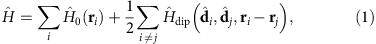where the sums run over all particles in the system and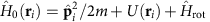is the single-particle Hamiltonian. Here, m is the mass of a molecule, U(r) is the external periodic potential and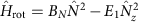is the Hamiltonian of the internal degrees of freedom giving the spectrum in Fig. 1b. The dipole–dipole interaction between two molecules i and j with dipole moments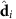andand separated by vector Rij (Fig. 1a) is given by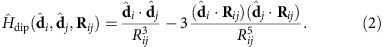Introducing polar coordinates Rij=(Rij, φij), the interaction Hamiltonian (2) can be decomposed as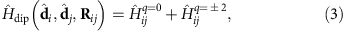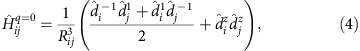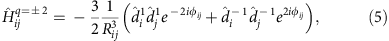whereare spherical components of the dipole operator of molecule j.

The operator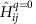, equation (4), conserves both the total internal angular momentum of the interacting molecule and its z-component. As shown in Fig. 1c,exchanges the states |1, ±1› and |0, 0› of two molecules while preserving Mi+Mj. Such ‘spin-exchange’ dipolar interactions have been observed in recent experiments on polar molecules7, Rydberg atoms27,28 and magnetic atoms29. In contrast, the operator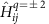transfers angular momentum between the internal and external orbital motion of the molecules, while preserving the total projection onto the z axis. Namely, the operator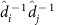decreases the internal angular momentum by 2, whileincreases the orbital angular momentum of a molecule by 2, thus preserving the total angular momentum (see, Fig. 1d). As we shall show, this transfer of angular momentum is responsible for the generation of the effective SOC of the elementary excitations.

### Phenomenological analysis

Let us assume that almost all the molecules are initially in their lowest rotational level |0, 0› and are in a spatially uniform, not necessarily equilibrium state, but with a relaxation time sufficiently long to be considered stationary. We then suppose that this state is slightly perturbed by a resonant microwave pulse, which excites a small number of molecules from |0, 0› to |1, ±1›. In what follows we show that the density and angular momentum dynamics of the |1, ±1› rotational levels after such excitation is equivalent to that of an ideal gas of spin-1/2 chiral quasiparticles (chirons).

The emergence of the excitations can be phenomenologically understood as follows. Due to the translational invariance of the Hamiltonian of the system, the (quasi)momentum k (in the presence of an optical lattice), with polar coordinates (k, φk) (Fig. 2a) is a good quantum number. In the long-wave limit k→0, there is a degeneracy between the excitations carrying molecules in the rotational states |↑›≡|1, 1› and |↓›≡|1, −1› due to the symmetry with respect to inverting the dipole moment. Note that the other excited rotational states are separated from |↑› and |↓› by large energy gaps and do not participate in the dynamics. This allows us to consider a reduced space {|↑›, |↓›} of rotational states. Each of these states can be obtained from the other by acting with the operators. Hence, the most general form of the excitation Hamiltonian in the reduced space reads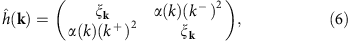where k±=kx±iky, and α(k) and ξk are some functions of k.

The Hamiltonian in equation (6) describes quasiparticles with a two-branch spectrum with energies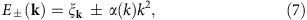corresponding to the eigenstates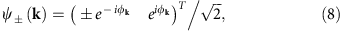respectively, and a Berry phase of 2π. In a system with an inversion-symmetric Hamiltonian, ĥ(k)=ĥ(−k), the Berry phase can be defined30 modulo 4π as an integralalong a contour C connecting two points k and −k in momentum space. Thus, the Berry phase 2π of chirons is non-trivial. As is necessary in a system with time-reversal symmetry31,32, ΦBP is a multiple of π.

Let us notice that if α(k)=const, the Hamiltonian (6) coincides with that of the low-energy excitations in bilayer graphene21,22 in the wavelength limit k→0. In the next section, we demonstrate that such Hamiltonian is indeed realised in the case of weak interactions between the molecules.

### Microscopic calculation of the Hamiltonian

In the previous section, we have shown phenomenologically that the effective Hamiltonian of long-wave excitations in a system of polar molecules has the form given by equation (6). In what immediately follows, we demonstrate that the Hamiltonian can be explicitly evaluated microscopically in the two opposite limits: when the kinetic energy is negligible compared with the characteristic interaction strength and when the interactions are small compared with the kinetic energy. While the first regime can be achieved by pinning the molecules in a deep optical lattice and is thus relevant for current experiments with reactive molecules7, the second regime could be in principle realised in the future with molecules that are non-reactive and less susceptible to überresonant processes33.

To address the first limit, we consider molecules in a deep, unit-filled square optical lattice. In this setting, the translational degrees of freedom are frozen and dynamics occurs only in the internal degrees of freedom. The dynamics can be mapped to that of a gas of bosons with spin σε{↑,↓} and long-range hopping. The vacuum corresponds to all molecules being in the |0, 0› state. The effective Hamiltonian describing the rotational excitations can be expressed in terms of the bosonic creation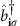and annihilation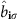operators of rotational excitations with σ character at lattice site i={ix, iy}, asHere, the hopping constants J0 and J2 are determined by dipole matrix elements. We work in the hard-core limit, which restricts the occupation number on each site to 0 or 1. The hard-core constraint encapsulates that there is at most one molecule per lattice site and each molecule can harbour at most one N=1 rotational excitation. Physically, the hard-core constraint can stem either from strong elastic interactions or rapid inelastic loss rates, for example, two-body chemical losses, at short range7,34.

The dispersions of a single rotational excitation are given byand shown in Fig. 2. Here, F(n)(k)=∑j≠0exp(−i k·rj+inφj)|rj|−3 with rj a vector connecting sites in the square lattice and φj the polar angle of rj. The phase ϕk of F(2), that is,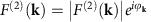, determines the polar angle of the Bloch vector (Fig. 2a). In the long-wave limit, k→0, we obtain in accordance with equation (6) that ϕk≈2φk, ξk/J0A+2π/k and α(k)=2πJ2/(3k), with A≈9.03. For general ratios J2/J0, both branches have a conical dispersion for small k. For the case J2=3J0, as results from the geometry in Fig. 1a, there is a cancellation of the linear k component in the E+ branch. This leads to a locally flat dispersion E+(k)/J0A+(k2) and a conical dispersion E(k)/J0A+4πk+(k2) (Fig. 2b).

In the case of a sufficiently shallow optical lattice or weak interactions, the kinetic energy of the molecules can dominate over the mean interaction energy. In this case, the dynamics can be analysed perturbatively in the interactions. By explicitly evaluating the Hamiltonian of the excitations, the details of which are provided in Supplementary Methods, we reproduce equation (6) with the off-diagonal entry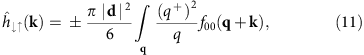where the upper and the lower signs apply to bosonic and fermionic molecules, respectively; f00(q) is the distribution function of the molecules in the |0, 0› state. It is assumed to be stationary and independent of the molecule position, but it is not restricted to be in thermal equilibrium; q+=qx+iqy; and ∫q…=∫(2π)−2d2q. Due to the smallness of the interactions, the diagonal elements of the matrix in equation (6) are close to the kinetic energy of a single molecule and are only slightly modified by the interactions, ξkk2(2m)−1 [1+(|d|2)]. This calculation is performed explicitly in Supplementary Methods.

The long-time dynamics is dominated by small momenta. For an isotropic distribution function f00(q)=f00(q), from equation (11) we find the value of the spin–orbital coupling in the limit k→0 to beFor a Fermi liquid of fermionic molecules at zero temperature [f00(q)=θ(kFq)], equation (12) yields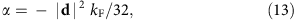where kF is the Fermi momentum. At sufficiently high temperatures Tn/m, where n is the density of the molecules (per nuclear spin), the distribution function is close to that of a Boltzmann gas,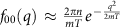, and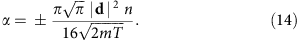For cold atoms in a quadratic trapping potential, the density of the molecules depends on temperature as n(T)T−1, resulting in the temperature dependency of the SOC α(T)T−3/2.

### Chirality manifestations in spin and density dynamics

The chirality of the excitations can be observed in the dynamics of the spin-1/2 operator, S={Ŝx, Ŝy, Ŝz}, in the reduced space of the rotational levels |↑› and |↓›. Let us assume that a short laser pulse excites a group of molecules in a small region of characteristic size Λ around r=0, |0, 0›→|↑› (the results of this paper can be easily generalized to include more general excitation protocols, |0, 0›→A|↑›+A|↓›). The internal state |↑› corresponds to excitation by light with right-circular polarization x+iy, and has a definite phase winding, as shown in Fig. 1b. Hence, for a spatially isotropic distribution of excitations, the laser polarization is what determines the spatial phase pattern emerging during the dynamics.

For sufficiently small Λ chirons leave the excited region quickly, reaching sufficiently low density, so that interactions between them can be neglected, and their dynamics is described by the kinetic equation for free particles, see Methods. In principle, chiron–chiron interactions may be important for hard-core particles in a deep optical lattice in the beginning of the dynamics, which, however, will not affect the results qualitatively. In the limit of pinned molecules, we additionally check whether chiron–chiron interactions can be neglected by numerically simulating the dynamics of two excitations, see Supplementary Methods and Supplementary Figs 2 and 3.

The chiral nature of the excitations is clearly visible in the density of Ŝx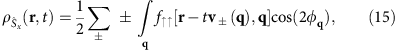where f↑↑(r, q) is the distribution function of the molecules in the |r, q› state at time t=0, v±(q) are the velocities of the two chiron branches and φq is the polar angle of the vector q. In the long-wave limit (see equation (7))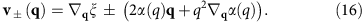Equation (15) describes the spin distribution at sufficiently long times, when it is dominated by long-wave chirons (qa−1). In this limit, the phase factor cos(2φq) originates from the off-diagonal element of equation (6). To account for arbitrary-momenta excitations, 2φq in equation (15) has to be replaced by the polar angle ϕq of a chiron state on the Bloch sphere (Fig. 2a). For the small Λ under consideration, the distribution has a d-wave symmetry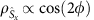at long times (Fig. 3a,b), which is a manifestation of the non-trivial Berry phase 2π of the excitations. The radial distribution of the spin component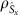after applying a narrow laser pulse depends on the molecular statistics, interaction strength, optical lattice depth and so on, while its d-wave symmetry is universal, being a consequence of the Berry phase 2π.

The spatial distribution of the spin coherences can be particularly easily understood in the case of a Fermi liquid (fermionic molecules at low temperatures) with weak interactions. In this case, the excitations propagate at the maximal speed υ+(kF), kF being the Fermi momentum. At a distance r away from the initial excitation pulse, the spin distribution remains unaltered until time t=r/υ+(kF) when it is reached by the quickest branch of chirons with the angular distribution of spins. At a slightly later moment of time t=r/υ(kF), the same point is reached by a wave of slower chirons with opposite spin, after which the spin density remains very small. The resulting distribution is shown in Fig. 3a.

In the case of a deep optical lattice, the branch of chirons with the dispersion E(q) is significantly faster than the other branch, leading to a quick spatial separation of the two branches (Fig. 3b) after applying the laser pulse. The outer circular density front corresponds to the faster chirons, which propagate at a nearly constant speed, while the more complex inner pattern comes from the slower branch of chirons. Despite different dispersions of the chirons, the angular distribution of the spin is again cos(2φ).

Because of the SOC, spin is not conserved and the total numbers of molecules in the rotational states |↑› and |↓›,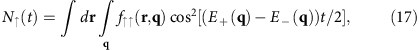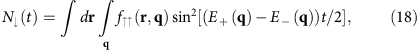are time dependent. At long times t→∞, both N(t) and N(t) saturate at a half of the number Nex of the initially excited molecules (Fig. 4c) regardless of the details of the distribution function f↑↑(r, q). The total number of molecules in excited rotational states is conserved, N(t)+N(t)=Nex.

Thus, the SOC transfers the internal angular momentum of the molecules, all of which are in the |↑› state at t=0, to their orbital motion, leading to the formation of a vortex structure around r=0. This manifests itself, for instance, in a dip in the density of molecules in the |↓› rotational state,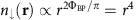, around the centre of the vortex structure (Fig. 4b). In particular, if the chiron spectrum has a branch with quadratic dispersion E(k)~k2(2M)−1, which is realised, for example, for weak interactions in a shallow optical lattice or for strong interactions in a deep optical lattice, the density of the spin-down molecules close to the centre of the vortex estimates n(r, t)~(M2r2/t2)2 Nex2 at sufficiently long times. Far from the centre of the vortex, the density profile is described by freely propagating chirons that do not interfere with each other: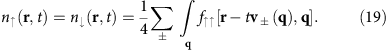The two contributions in the sum in equation (19) correspond to the two branches of chirons propagating with velocities υ+(q) and υ(q), which leads to their spatial separation.

### Experimental accessibility

In this section, we discuss some details related to the observation of dipolar SOC in present cold polar molecule experiments, taking as a representative example the KRb experiment at JILA7. To prevent chemical reactions, KRb polar molecules are pinned in a deep 3D optical lattice. The relevant energy scales for equation (9) are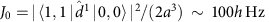and J2=3J0. Trapping in a deep optical lattice may also be required for molecular species that are chemically stable, as the presence of a very high density of resonances at ultracold energies has been proposed to lead to long-lived collision complexes, which are highly susceptible to three-body loss33.

The chirons’ spectra in the entire Brillouin zone can be measured by means of Rabi spectroscopy provided the probe beam can transfer the required quasi-momentum kR1/a to the molecules. Direct microwave transitions are insufficient since they have kRa1, but kRa1 can be achieved using optical Raman pulses. Here, kR=|k1k2|, with ki the wavevector of the ith Raman beam. Raman transitions between internal states are already a key part of the production of ground-state molecules through STIRAP35; our proposal requires only minor modifications of this well-established procedure.

Due to the inherent difficulty of directly cooling molecules, present experiments are not quantum degenerate, leading to a sparse lattice-filling fraction near 10%7,8,34. Figure 3c displays the density of Ŝx in a lattice with 10% filling, averaged over disorder realizations. The d-wave symmetry of the distribution is still visible, albeit with reduced contrast compared with the unit-filled case (Fig. 3b). The d-wave symmetry is a consequence of the Berry phase, a topological property, and so is robust against disorder. In contrast, disorder smears the vortex structure.

In the rotational structure of KRb, nuclear quadrupole interactions cause the states with predominant |1, −1› and |1, 1› character to be non-degenerate by about 70 hkHz at the 545 G magnetic fields used for magneto-association36. These states can be made degenerate and out of resonance with the |1, 0› level by increasing the strength of the magnetic field B, even at zero electric field. In this scenario, the B field determines the quantization axis. For 40K87Rb, where the nuclear quadrupole moments are (eqQ)Rb=−1.380 hMHz and (eqQ)K=0.452 hMHz36, the levels cross near B1,260 G, well within experimental feasibility. The energy difference between these levels close to the crossing is nearly linear, with a slope of roughly 40 Hz G−1. Stabilization of magnetic fields at the 10 mG level, which is routine in ultracold gas experiments, would correspond to non-degeneracy on the order of 0.4 Hz, and will not significantly affect our results. Similar comments apply for the other alkali metal dimers. The level structure in Fig. 1b also results for 1Σ molecules without hyperfine structure, for example, bosonic SrO, in the presence of a uniform electric field.

Finally, we note that several knobs can be used to manipulate the Hamiltonian, equation (9). For example, by changing the angle of the quantization axis with respect to the space-fixed z axis, one can tune the ratio J2/J0 and remove the cancellation seen in the E+ branch. This can be used in turn to control the propagation velocity of the two branches of chirons. Chiron–chiron interactions that fall off as 1/r3 can also be controllably introduced by turning on an external static electric field.

## Discussion

We have demonstrated that a 2D system of polar molecules behaves as a gas of chiral excitations with a Berry phase 2π. We have shown that signatures of those excitations, which resemble the low-energy excitations exhibited by bilayer graphene, manifest in both the dynamics of the density and spin coherences.

The implementation of SOC in polar molecules presented here can open other exciting research avenues. In particular, by superimposing an effective magnetic field to the chirons via light-generated synthetic gauge fields23, it might be possible to simulate the unconventional quantum Hall effect of bilayer graphene and to see its intriguing consequences21 in the lowest Landau levels in the limit of low chiron density and high synthetic magnetic fields. Such an effect would require fermionic statistics of the excitations, which can be realised with fermionic molecules in a shallow optical lattice.

Although so far the system in consideration is an ideal or nearly ideal gas of chirons and interactions between them can be neglected, chiron–chiron interactions tunable by the duration of the laser pulse, the size of the excited region or an external electric field may lead to very rich physics. For example, chiron–chiron interactions together with non-stationary background N=0 molecules or microwave dressing can give rise to interesting dynamic structures, new types of transport phenomena and even to fractional quantum Hall phases when combined with synthetic gauge fields13,37.

Finally, we expect our predictions to be extendable to other dipole–dipole interacting systems such as Rydberg atoms, magnetic atoms and magnetic defects in solids.

## Methods

### Kinetic equation for polar molecules

To characterize the dynamics of a system of polar molecules, we introduce the non-equilibrium Green’s functions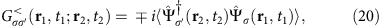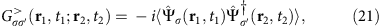where σ and σ′ label the internal states of the molecules (σ corresponds to (|1, −1›, |0, 0›, |1, 1›)). The upper (lower) sign applies to bosonic (fermionic) particles.

The distribution functions of the molecules are defined as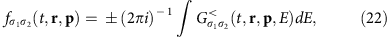where the upper and the lower signs apply to bosonic and fermionic particles, respectively, and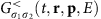is the result of the Wigner transformation38,39 of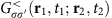.

Using the functions (20) and (21), we define a 2 × 2 matrix in the Keldysh space38,39,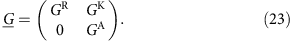Each of the Green’s functions in equation (23) is a matrix in the space of the internal rotational levels of the molecules.

The function (23) satisfies the equation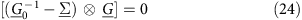(Dyson equation minus its conjugate), where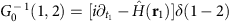; 1=(t1, r1), 2=(t2, r2) and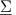is the self-energy part, determined by the dipole–dipole interactions.

In terms of the distribution functions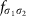, the kinetic equation reads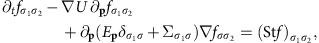where U(r) is the external smooth (trapping) potential, Ep is the kinetic (quasi)energy, the summation over repeated indices is implied, and Stf is the collision integral, which accounts for the relaxation of the distribution function due to molecular collisions.

In this paper, we consider a model with a small relaxation rate, which can be neglected on the characteristic times of interest, so that the excitations propagate ballistically. Also, we assume that chirons reach sufficiently low density shortly after they are excited, so that chiron–chiron interactions can be neglected, and the problem becomes effectively single particle.

Introducing the chiron annihilation operator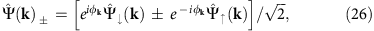where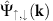are the annihilation operators for the plane-wave states in the reduced space {|↓›, |↑›} of the rotational levels of the molecules and the kinetic equation (25) is reduced, under the assumptions made above, to that for a single-particle problem with the dispersion E±(k):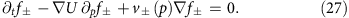In the case U=0, considered in this paper, the most general solution of equation (27) readsG±(r, p) being arbitrary functions of two arguments.